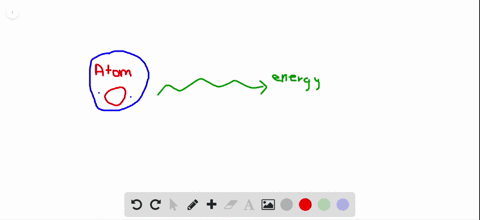### MAIN Idea Explain how unstable atoms gain stabili…

00:22University of Miami
Problem 24

Calculate Three magnesium isotopes have atomic masses and relative abun-
dances of 23.985 amu $(79.99 \%), 24.986$ amu $(10.00 \%),$ and 25.982$(11.01 \%) .$
Calculate the atomic mass of magnesium.

## Discussion

You must be signed in to discuss.

## Video Transcript

you know, work on problem 24 from chapter four in this problem, whereas, to calculate the atomic mass of magnesium provided, um, the atomic masses and relative abundance for three, uh, common magnesium face it tooks. So we do this by multiplying the abundance times the isotope mass and adding up for each isotope. So again, for, uh, for the abundance were given in percentage we want to use as a decimal. So you divide by 100. So 79.99% become 0.7999 would multiply it by the ah mass of that isotope, which is 23.985 Then we add this to another isotope which has a 10% abundance, which is 0.10 Uh, at the desk boom, we multiply by the massive this isotope just 24.986 and then finally, the third isotope has an abundance of 11.1% which become 0.1101 This isotope has massive 25.982 And so we multiply and add up here and we get an atomic mass of 24.54 atomic mass units for B uh, atomic man